1. /
2. CBSE
3. /
4. Class 06
5. /
6. Mathematics
7. /
8. NCERT Solutions for Class...

# NCERT Solutions for Class 6 Maths Exercise 6.1

NCERT solutions for Maths Integers## NCERT Solutions for Class 6 Maths Integers

###### Question 1.Write opposite of the following:

(a) Increase in weight

(b) 30 km north

(c) 326 BC

(d) Loss of Rs. 700

(e) 100 m above sea level

(a) Decrease in weight

(b) 30 km south

(d) Profit of Rs. 700

(e) 100 m below sea level

NCERT Solutions for Class 6 Maths Exercise 6.1

###### Question 2.Represent the following numbers as integers with appropriate signs.

(a)An aeroplane is flying at a height two thousand meters above the ground.

(b)A submarine is moving at a depth eight thousand meters below the sea level.

(c)A deposit of rupees two hundred.

(d)Withdrawal of rupees seven hundred.

(a) (+) 200 meters

(b) (–) 800 meters

(c) (+) 200 Rupees

(d) (–) 700 Rupees

###### Question 3.Represent the following numbers on number line:

(a) +5

(b) –10

(c) +8

(d) –1

(e) –6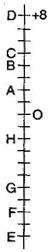(a)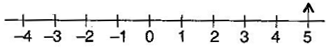(b)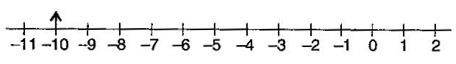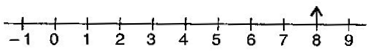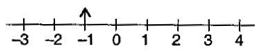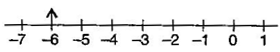NCERT Solutions for Class 6 Maths Exercise 6.1

###### Question 4.Adjacent figure is a vertical number line, representing integers. Observe it and locate the following points:

(a)If point D is +8 then which point is –8?

(b)Is point G a negative integer or a positive integer?

(c)Write integers for points B and E.

(d)Which point marked on this number line has the least value?

(e)Arrange all the points in decreasing order of values.

(a) F

(b) Negative

(c) B = (+) 4; E = (–) 10

(d) E

(e) D, C, B, A, O, H, G, F, E

###### Question 5.Following is the list of temperatures of five places in India, on a particular day of the year.
 Place Temperature Siachin 10{tex}{}^\circ {/tex} C below 0{tex}{}^\circ {/tex}C _______________________ Shimla 2{tex}{}^\circ {/tex}C below 0{tex}{}^\circ {/tex}C _______________________ Ahmedabad 30{tex}{}^\circ {/tex}C above 0{tex}{}^\circ {/tex}C _______________________ Delhi 20{tex}{}^\circ {/tex}C above 0{tex}{}^\circ {/tex}C _______________________ Srinagar 5{tex}{}^\circ {/tex}C below 0{tex}{}^\circ {/tex}C _______________________

(a)Write the temperature of these places in the form of integers in the blank column.

(b)Following is the number line representing the temperature in degree Celsius.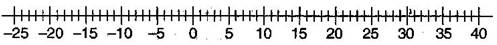Plot the name of the city against its temperature.

(a)Which is the coolest place?

(b)Write the names of the place where temperature are above 10oC.

(a)

 Place Temperature Siachen (-) 10{tex}{}^\circ {/tex}C Shimla (–) 2{tex}{}^\circ {/tex}C Ahmedabad (+) 30{tex}{}^\circ {/tex}C Delhi (+) 20{tex}{}^\circ {/tex}C Srinagar (–) 5{tex}{}^\circ {/tex}C

(b)Number line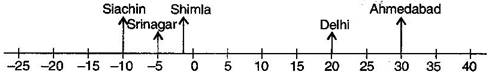(c)Siachin

NCERT Solutions for Class 6 Maths Exercise 6.1

###### Question 6.In each of the following pairs, which number is to the right of the other on the number line?

(a) 2, 9

(b) –3, –8

(c) 0, –1

(d) –11, 10

(e) –6, 6

(f) 1, –100

(a) 9 is right to 2

(b) –3 is right to –8

(c) 0 is right to –1

(d) 10 is right to –11

(e) 6 is right to –6

(f) 1 is right to –100

###### Question 7.Write all the integers between the given pairs (write them in the increasing order):

(a) 0 and –7

(b) –4 and 4

(c) –8 and –15

(d) –30 and –23

(a) –6, –5, –4, –3, –2, –1

(b) –3, –2, –1, 0, 1, 2, 3

(c) –14, –13, –12, –11, –10, –9

(d) –29, –28, –27, –26, –25, –24

NCERT Solutions for Class 6 Maths Exercise 6.1

###### Question 8.

(a) Write four negative integers greater than –20.

(b) Write four negative integers less than –10.

(a) –19, –18, –17, –16

(b) –11, –12, –13, –14

###### Question 9.For the following statements write True (T) or False (F). If the statement is false, correct the statement:

(a)–8 is to the right of –10 on a number line.

(b)–100 is the right of –50 on a number line.

(c)Smallest negative integer is –1.

(d)–26 is larger than –25.

(a) True

(b) False

(c) False

(d) False

NCERT Solutions for Class 6 Maths Exercise 6.1

###### Question 10.Draw a number line and answer the following:

(a)Draw a number line will we reach if we move 4 numbers to the right of –2.

(b)Which number will we reach if we move 5 numbers to the left of 1.

(c)If we are at –8 on the number line, in which direction should we move to reach –13?

(d)If we are at –6 on the number line, in which direction should we move to reach –1?

(a)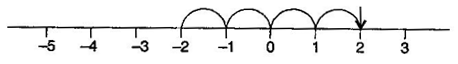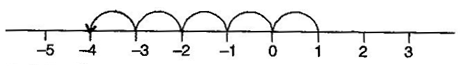(c) On left side

(d) On right side

## NCERT Solutions for Class 6 Maths Exercise 6.1

NCERT Solutions Class 6 Maths PDF (Download) Free from myCBSEguide app and myCBSEguide website. Ncert solution class 6 Maths includes text book solutions from Class 6 Maths Book . NCERT Solutions for CBSE Class 6 Maths have total 14 chapters. 6 Maths NCERT Solutions in PDF for free Download on our website. Ncert Maths class 6 solutions PDF and Maths ncert class 6 PDF solutions with latest modifications and as per the latest CBSE syllabus are only available in myCBSEguide.

## CBSE app for Students

To download NCERT Solutions for Class 6 Maths, Social Science Computer Science, Home Science, Hindi English, Maths Science do check myCBSEguide app or website. myCBSEguide provides sample papers with solution, test papers for chapter-wise practice, NCERT solutions, NCERT Exemplar solutions, quick revision notes for ready reference, CBSE guess papers and CBSE important question papers. Sample Paper all are made available through the best app for CBSE students and myCBSEguide website.Test Generator

Create question paper PDF and online tests with your own name & logo in minutes.myCBSEguide

Question Bank, Mock Tests, Exam Papers, NCERT Solutions, Sample Papers, Notes